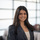# Transposing Tables using Python Pandas

Pandas makes transposing tables beautifully simple! Below are a couple examples using the example SQL output below: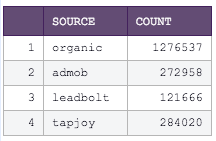This first Python snippet allows you to define your own column headers:

```# SQL output is imported as a pandas dataframe variable called "df"
import pandas as pd

df2=df.T
df2.columns=['label 1', 'label 2','label 3','label 4']

# Use Periscope to visualize a dataframe or an image by passing data to periscope.output()
periscope.output(df2)```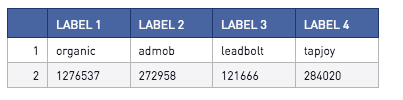And this Python snippet makes the first row your column headers

```# SQL output is imported as a pandas dataframe variable called "df"
import pandas as pd
df2=df.T
df2=df2[1:]
# Use Periscope to visualize a dataframe or an image by passing data to periscope.output()
periscope.output(df2)```Interested in learning about pivoting tables in Python or R? Check out the post here for more information.

Prefer R? Check out the community post here!

• Oldest first
• Popular•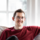Transposing back to the original table is a little different!

Suppose your SQL output is something like this:And you wanted your output to look like this: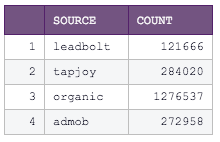In this case, you'd need to reset the index to preserve the original column titles as well as rename the resulting columns. The following Python code will do nicely!

```# SQL output is imported as a pandas dataframe variable called "df"
import pandas as pd

df = df.T.reset_index()
df.columns = ["source", "count"]

# Use Periscope to visualize a dataframe or an image by passing data to periscope.output()
periscope.output(df)

```